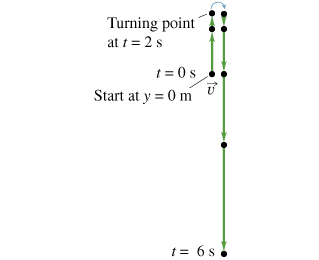# Problem: A stone is thrown upward from the edge of a cliff, reaches its maximum height, and then falls down into the valley below. A motion diagram for this situation is given (Figure 1) , beginning the instant the stone leaves the thrower’s hand.Construct a graph corresponding to the stone's vertical velocity, vy(t), taking the acceleration due to gravity as exactly 10 m/s2. Ignore air resistance. The unit of time is in seconds and the unit of displacement is in meters. In plotting the points, round-off the coordinate values to the nearest integer.

###### FREE Expert Solution

UAM expressions:

V0 = 20 m/s

At t = 0 s, v0 = (20) - (10)(0) = 20 m/s

At t = 2 s, v0 = (20) - (10)(2) = 0 m/s

At t = 4 s, v0 = (20) - (10)(4) = -20 m/s

At t = 6 s, v0 = (20) - (10)(6) = -40 m/s

83% (63 ratings)###### Problem Details

A stone is thrown upward from the edge of a cliff, reaches its maximum height, and then falls down into the valley below. A motion diagram for this situation is given (Figure 1) , beginning the instant the stone leaves the thrower’s hand.

Construct a graph corresponding to the stone's vertical velocity, vy(t), taking the acceleration due to gravity as exactly 10 m/s2. Ignore air resistance. The unit of time is in seconds and the unit of displacement is in meters. In plotting the points, round-off the coordinate values to the nearest integer.